# Class 12 Maths NCERT Solutions for Chapter 9 Differential Equations Exercise 9.1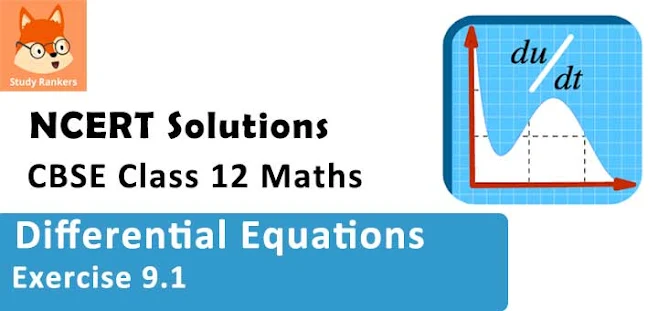### Differential Equations Exercise 9.1 Solutions

1. Determine order and degree (If defined) of differential equation d4y /dx4 + sin(y')  = 0

Solution

d4y /dx4 + sin(y''')  = 0
⇒ y''' + sin(y''') = 0
The highest order derivative present in the differential equation is y''''. Therefore, its order is four.
The given differential equation is not a polynomial equation in its derivatives. Hence, its degree is not defined.

2. Determine order and degree(if defined) of differential equation y' + 5y = 0

Solution

The given differential equation is :
y' + 5y = 0
The highest order derivative present in the differential equation is y' . Therefore, its order is one.
It is a polynomial equation in y'. The highest power raised to y' is 1. Hence, its degree is one.

3. Determine order and degree (if defined of differential equation (ds/dt)4 + 3s d2s/dt2 = 0

Solution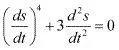The highest order derivative present in the given differential equation is d2s/dt2. Therefore, its order is two .
It is a polynomial equation in d2s/dt2  and ds/dt. The power raised to d2s/dt2 is 1.
Hence, its degree is one.

4. Determine order and degree (if defined) of differential equation d2y/(ds2)2 + cos (dy/dx) = 0
Solution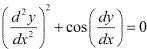The highest order derivative present in the given differential equation is d2 y/dx2. Therefore, its order is 2.
The given differential equation is not a polynomial equation in its derivatives. Hence, its degree is not defined.

5. Determine order and degree (if defined) of differential equation d2 y/dx2 = cos 3x + sin 3x
Solution
d2y/dx2 = cos 3x + sin 3x
⇒ d2y/dx2 - cos 3x - sin 3x = 0
The highest order derivative present in the differential equation is d2y/dx2 . Therefore, its order is two.
It is a polynomial equation in d2 y/dx2 and the power raised to d2y/dx2 is 1.
Hence, its degree is one.

6. Determine order and degree (if defined) of differential equation
(y''') + (y'')3 + (y')4 + y5 = 0
Solution
(y''') + (y'')3 + (y')4 + y5 = 0
The highest order derivative present in the differential equation is y'''. Therefore, its order is three.
The given differential equation is a polynomial equation in y''' , y'' , and y' .
The highest power raised to y''' is 2. Hence, its degree is 2.

7. Determine order and degree(if defined) of differential equation y′′′ + 2y″ + y′ = 0
Solution
y′′′ + 2y″ + y′ = 0
The highest order derivative present in the differential equation is y'''. Therefore, its order is three.
It is a polynomial equation in y''', y'' and y' . The highest power raised to y''' is 1. Hence, its degree is 1.

8. Determine order and degree(if defined) of differential equation y′ + y = ex  .
Solution
y′ + y = ex
⇒ y' + y - ex  = 0
The highest order derivative present in the differential equation is y'. Therefore, its order is one.
The given differential equation is a polynomial equation in y' and the highest power raised to y' is one. Hence, its degree is one.

9. Determine order and degree (if defined) of differential equation y'' + (y')2 + 2y = 0
Solution
y'' + (y')2 + 2y = 0
The highest order derivative present in the differential equation is y''. Therefore, its order is two.
The given differential equation is a polynomial equation in y'' and y' and the highest power raised to y'' is one.
Hence, its degree is one .

10. Determine order and degree(if defined) of differential equation y″ + 2y′ + sin y = 0
Solution
y'' + 2y' + sin y = 0
The highest order derivative present in the differential equation is y''. Therefore, its order is two.
This is a polynomial equation in y'' and y' and the highest power raised to y'' is one.
Hence, its degree is one.

11. The degree of the differential equation
(d2y/dx2 )3 + (dy/dx)2 + sin(dy/dx) + 1 = 0
(A) 3
(B) 2
(C) 1
(D) not defined
Solution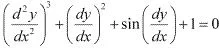The given differential equation is not a polynomial equation in its derivatives. Therefore, its degree is not defined.
Hence, the correct answer is D.

12. The order of the differential equation  2x2(d2y/dx2) + 3(dy/dx) + y = 0 is
(A) 2
(B) 1
(C) 0
(D) not defined
Solution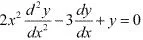The highest order derivative present in the given differential equation is d2 y/dx2 . Therefore, its order is two.
Hence, the correct answer is A.